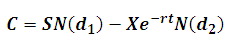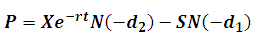# Black-Scholes-Merton (BSM) Option Pricing Model

• Commonly called "Black-Scholes" outside the CFA exam world.

• BSM is a model for deriving the price of an option.

• Assumptions

• Stock returns are lognormally distributed.

• The risk free rate is known and stays constant during the option term.

• The stock's volatility is known and stays constant during the option term.

• Transaction costs are omitted from the model.

• Dividends are excluded.

• The options cannot be exercised until expiration (i.e. the option is European).

• Weaknesses in BSM Assumptions

• It is good for candidates to understand that BSM is not without its flaws; it is after all a theoretical model.

• The risk free rate is not always "known" and may not stay constant for the option's life; further an investor is unlikely to be able to borrow at the risk free rate.

• A stock's volatility is not likely to remain constant over the life of the option.

• In reality, investors face transaction costs.

• Many stocks pay dividends.

• The investor may be trading American options and not European style options.

• BSM Formula:

Using the Black-Scholes model, the price of a call option is calculated using the following formula:Where: C is the price of the call option S is the price of the underlying stock X is the option exercise price r is the risk-free interest rate T is the current time until expiration N() is the area under the normal curve d1 =  [ ln(S/X) + (r + σ2/2) T ] / σ T1/2 d2 =  d1 - σ T1/2

Similarly, the price of the put option is:The CFAI may include the BSM formula as part of the candidate body of knowledge, so technically, it could be required on the exam. However, given the time constraints of the exam, calculation of the BSM formula is unlikely.

• Candidates are encouraged to focus on understanding the BSM and its applications (especially the "Greeks"), rather than the formula itself.

## Underlying Asset Cash Flows and Option Prices

• While BSM assumes that the underlying asset does not have any cash flows, in reality many stocks pay dividends.
• Holding other variables constant, dividend payments reduce the value of calls and increase the value of puts. This is because stock prices are adjusted down once the stock goes ex-dividend.
• BSM can be adjusted for a dividend payment by reducing the spot price (S0) of the stock by the present value of the dividend payment expected to take place during the option's life.
• For stock's a continuously compounded dividend yield can represent the cash flow; for currencies, a continuously compounded interest rate can be applied.